# Pictures

Jump to: navigation, search

A class of bijections (cf. Bijection) between subsets of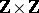, namely skew diagrams. A skew diagram is a finite subset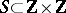such that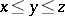withimplies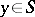, where "≤" is the coordinatewise partial ordering of; a typical skew diagram is the difference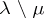of two Young diagrams (cf. Young diagram)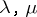with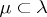. The definition of pictures also uses another partial ordering "≤" on, given by(sometimes the opposite ordering is used instead); a bijectionbetween two skew diagrams is a picture if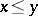implies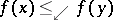and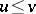implies. The set of all pictures has various symmetries, among which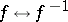.

When domain and image are fixed to certain shapes, pictures become equivalent to many other combinatorial concepts, such as permutations, (semi-) standard Young tableaux, skew tableaux, Littlewood–Richardson fillings, and matrices overorwith prescribed row and column sums. On the other hand, any picture gives rise to a semi-standard skew tableau by projecting its images onto their first coordinate. For any skew diagrams,, the number of pictures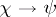is equal to the intertwining number of representations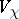and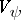of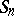, or of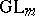, see [a5]. In particular, the number of pictures fromto, for Young diagrams,,, is the multiplicity of the irreducible representation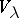ofin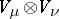; this is essentially the Littlewood–Richardson rule.

There is a natural bijection between pictures, for arbitrary skew shapes,, and pairs of pictures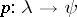and, for some Young diagram. This is a generalization of the Robinson–Schensted correspondence, and it agrees with the intertwining number interpretation. It also gives a decomposition of skew Schur polynomials into ordinary Schur polynomials, generalizing the decomposition of the character ofmentioned in Robinson–Schensted correspondence, and thereby provides a proof of the Littlewood–Richardson rule; this is closely related to the reason that correspondence was originally introduced in [a3]. Like the-symbol in the ordinary Robinson–Schensted correspondence, the picturecan not only be computed fromby an insertion procedure, but also by using the jeu de taquin (see [a4]), to gradually transform the domaininto a Young diagram. By the symmetry, the picturecan also be computed by the jeu de taquin at the image side, to transform the imageinto. The steps of these two forms of the jeu de taquin commute with each other, and this provides a key to many properties of the Robinson–Schensted correspondence [a2].